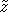Name
• String
• Substring
• Search
Edit

The pattern x is factorized in two parts xand xr such that x=xxr. Then the search phase of the Two Way algorithm consists in comparing the characters of xr from left to right and then, if no mismatch occurs during that first stage, in comparing the characters of xfrom right to left in a second stage.

The preprocessing phase of the algorithm consists then in choosing a good factorization  xxr.

Definition

Let (uv) be a factorization of x. A repetition in (uv) is a word w such that the two following properties hold:

• w is a suffix of u or u is a suffix of w;
• w is a prefix of v of v is a prefix of w.

In other words w occurs at both sides of the cut between u and v with a possible overflow on either side. The length of a repetition in (u,v) is called a local period and the length of the smallest repetition in (u,v) is called the local period and is denoted by r(u,v).

Each factorization (u,v) of has at least one repetition. It can be easily seen thatr(u,v|x|

A factorization (u,v) of x such that r(u,v)=per(x) is called a critical factorization of x.

If (u,v) is a critical factorization of x then at the position |u| in x the global and the local periods are the same. The Two Way algorithm chooses the critical factorization (x,xr) such that |x| < per(x) and |x| is minimal.

To compute the critical factorization x,xr of x we first compute the maximal suffix z of x for the orderand the maximal suffixfor the reverse order. Then (x,xr) is chosen such that. |x|=max{|z|,||}

The preprocessing phase can be done in O(m) time and constant space complexity.

The searching phase of the Two Way algorithm consists in first comparing the character of xr from left to right, then the character of xfrom right to left.

When a mismatch occurs when scanning the k-th character of xr, then a shift of length k is performed.

When a mismatch occurs when scanning x, or when an occurrence of the pattern is found, then a shift of length per(x) is performed.

Such a scheme leads to a quadratic worst case algorithm, this can be avoided by a prefix memorization: when a shift of length per(x) is performed the length of the matching prefix of the pattern at the beginning of the window (namely m-per(x)) after the shift is memorized to avoid to scan it again during the next attempt.

The searching phase of the Two Way algorithm can be done in O(n) time complexity.

The Two Way algorithm performs 2n-m text character comparisons in the worst case. Breslauer designed a variation on the Two Way algorithm which performs less than 2n-m comparisons using constant space.

Main features:

• requires an ordered alphabet;
• preprocessing phase in O(m) time and constant space complexity;
• constant space complexity for the preprocessing phase;
• searching phase in O(n) time;
• performs 2n-m text character comparisons in the worst case.

Example:

Preprocessing phaseFactorisation used by Two Way algorithm.

Searching phase

 G C A T C G C A G A G A G T A T A C A G T A C G 1 2 G C A G A G A G

Shift by: 2

 G C A T C G C A G A G A G T A T A C A G T A C G 1 G C A G A G A G

Shift by: 1

 G C A T C G C A G A G A G T A T A C A G T A C G 1 G C A G A G A G

Shift by: 1

 G C A T C G C A G A G A G T A T A C A G T A C G 1 G C A G A G A G

Shift by: 1

 G C A T C G C A G A G A G T A T A C A G T A C G 7 8 1 2 3 4 5 6 G C A G A G A G

Shift by: 7

 G C A T C G C A G A G A G T A T A C A G T A C G 1 2 G C A G A G A G

Shift by: 2

 G C A T C G C A G A G A G T A T A C A G T A C G 1 2 G C A G A G A G

Shift by: 2

 G C A T C G C A G A G A G T A T A C A G T A C G 1 2 3 G C A G A G A G

Shift by: 3

The Two Way algorithm performs 20 character comparisons on the example.

C